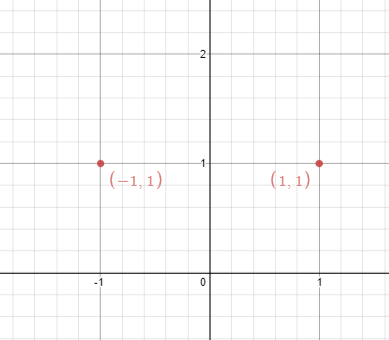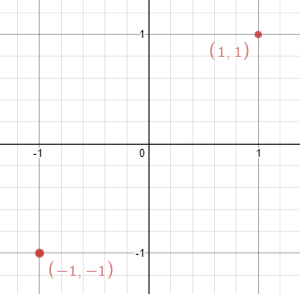356. Line Reflection

Given n points on a 2D plane, find if there is such a line parallel to y-axis that reflect the given points symmetrically, in other words, answer whether or not if there exists a line that after reflecting all points over the given line the set of the original points is the same that the reflected ones.

Note that there can be repeated points.

Could you do better than O(n2) ?

Example 1:```Input: points = [[1,1],[-1,1]]
Output: true
Explanation: We can choose the line x = 0.
```

Example 2:```Input: points = [[1,1],[-1,-1]]
Output: false
Explanation: We can't choose a line.
```

Constraints:

• `n == points.length`
• `1 <= n <= 10^4`
• `-10^8 <= points[i][j] <= 10^8`

356. Line Reflection
``````struct Solution;

use std::collections::HashSet;

impl Solution {
fn is_reflected(points: Vec<Vec<i32>>) -> bool {
let min = points.iter().map(|v| v).min().unwrap();
let max = points.iter().map(|v| v).max().unwrap();
let x = max + min;
let mut hs: HashSet<(i32, i32)> = HashSet::new();
for v in &points {
hs.insert((v, v));
}
for v in &points {
if !hs.contains(&(x - v, v)) {
return false;
}
}
true
}
}

#[test]
fn test() {
let points = vec_vec_i32![[1, 1], [-1, 1]];
let res = true;
assert_eq!(Solution::is_reflected(points), res);
let points = vec_vec_i32![[1, 1], [-1, -1]];
let res = false;
assert_eq!(Solution::is_reflected(points), res);
}
``````